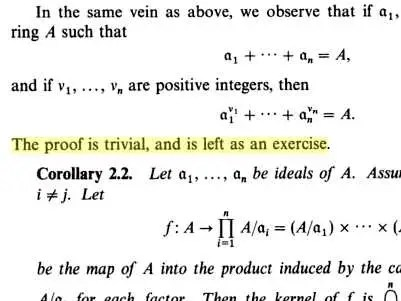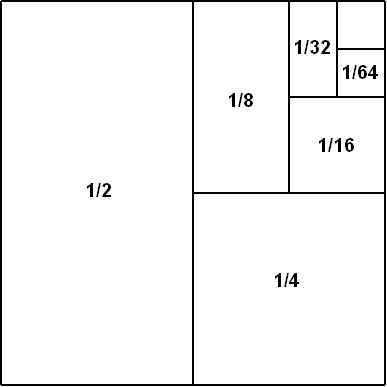# 13 Math Jokes That Every Math Geek Will Find Hilarious

Back when the internet was young, the primary users were its builders, math and tech-oriented academics spread around the country.

As a result, math jokes have an elemental role in the history of the internet.

From the earliest Usenet threads to the techiest subreddits, geeky math jokes — some implicit swipes at less-pure disciplines, other puns or plays on words of different concepts — have been a major part of the modern history of math.

What’s more, these japes also have the effect of making those who didn’t get the joke to look into what makes it funny, teaching people some of the more obscure concepts.

Here are just a few of the best ones. Where necessary, we’ll do the unthinkable and the tacky and explain the joke.

### JOKE #1

Three statisticians go out hunting together. After a while they spot a solitary rabbit. The first statistician takes aim and overshoots. The second aims and undershoots. The third shouts out “We got him!”

Source: chjilloutdamnit / Reddit

### JOKE #2

Two random variables were talking in a bar. They thought they were being discrete but I heard their chatter continuously.

Source: armchairdetective /  reddit

Explanation: When you roll a die, you either get a 1, 2, 3, 4, 5, or 6. Since there are a finite number of possibilities, the statistic involved is called a discrete random variable. When you select any real number from between 0 and 1, there are an infinite number of possible draws. The statistic involved is called a continuous random variable.

### JOKE #3

There was a statistician that drowned crossing a river… It was 3 feet deep on average.

Source: anatiferous_outlaw / reddit246-You / Flickr

### JOKE #4

Write the expression for the volume of a thick crust pizza with height “a” and radius “z”.

Source: Reddit

Explanation: The formula for volume is π·(radius)2·(height). In this case, pi·z·z·a.

### JOKE #5

A: “What is the integral of 1/cabin?”

B: “log cabin.”

A: “Nope, houseboat–you forgot the C.”

Source: Reddit

Explanation: We’re treating “cabin” is a variable.

The integral of 1/x is loge(x).

However, since it’s integration, you’ve got to add a constant.

So ∫(1/cabin) = loge(cabin) + c, or “a log cabin plus the sea.”

### JOKE #6Algebra. By Serge Lang

Q: Why did the chicken cross the road?

A: The answer is trivial and is left as an exercise for the reader.

Source: Reddit

Explanation:

This is a common refrain found in mathematics texts.

It is widely considered a cruel professor’s malicious cop-out by particularly lazy students of mathematics.

### JOKE #7

Q: How many mathematicians does it take to change a light bulb?

A: One: she gives it to three physicists, thus reducing it to a problem that has already been solved.

Source: MathOverflow

Explanation: Mathematicians try to reduce an unsolved problem to a form which has already been solved before. Once that’s done it’s considered complete, as the previously derived formula is taken as written.

There are many light bulb jokes about physicists. Finding several are left as an exercises to the reader.

### JOKE #8

A physicist, a biologist, and a mathematician are sitting on a bench across from a house. They watch as two people go into the house, and then a little later, three people walk out.

The physicist says, “The initial measurement was incorrect.”

The biologist says, “They must have reproduced.”

And the mathematician says, “If exactly one person enters that house, it will be empty.”

Source: Reddit

### JOKE #9

The B in Benoît B. Mandelbrot stand for Benoît B. Mandelbrot.

Source: Reddit

Explanation: The Mandelbrot set is a fractal. As you zoom in on portions of the fractal, you ee a self replicating image. So the infinite paradox in the joke is a shoutout to the problem. Here’s an example of what we’re talking about with a gif of zooming in on a point of infinite complexity in the Mandelbrot set:### JOKE #10Wikipedia

Infinitely many mathematicians walk into a bar. The first says, “I’ll have a beer.” The second says, “I’ll have half a beer.” The third says, “I’ll have a quarter of a beer.” The barman pulls out just two beers. The mathematicians are all like, “That’s all you’re giving us? How drunk do you expect us to get on that?” The bartender says, “Come on guys. Know your limits.”

Source: Reddit

Explanation: This is a reference to a converging infinite series.

The limit of this:

from n=0 to ∞   Σ (1/2n) = 1 + 1/2 + 1/4 + 1/8 + …  = 2

### JOKE #11

An infinite number of mathematicians walk into a bar. The first one orders a beer. The second orders half a beer. The third orders a third of a beer. The bartender bellows, “Get the hell out of here, are you trying to ruin me?”

Source: Reddit

Explanation: This is another hilarious reference to an infinite series — the harmonic series — which is not convergent but instead diverges to infinity.

from n=1 to ∞   Σ (1/n) = 1 + 1/2 + 1/3 + 1/4 + …  =

See a full explanation in this slideshow >

### JOKE #12

When a statistician passes the airport security check, they discover a bomb in his bag. He explains. “Statistics shows that the probability of a bomb being on an airplane is 1/1000. However, the chance that there are two bombs at one plane is 1/1000000. So, I am much safer…”

Source: Andrej and Elena Cherkaev

Explanation: While this statistician is correct that the joint probability there are two bombs on a plane is 1/1,000,000, his bringing one on doesn’t change the prior probability that there is still a 1/1,000 chance of his flight being the one with a random bomb.

### JOKE #13

What do you get when you cross a mosquito with a mountain climber?

Nothing. You can’t cross a vector and a scalar.

Source: Reddit

Explanation: A vector is a mathematical entity with both magnitude and direction in any number of dimensions. You can take the cross product of two vectors to form a new vector, similar to multiplication of real numbers.

A scalar is just a real number, a directionless magnitude in vector space. You cannot take a cross product of a scalar and a vector.

Hence, you can’t cross a mosquito (disease vector) and a mountain climber (a scalar).

That is one terrible pun. I’m sorry.